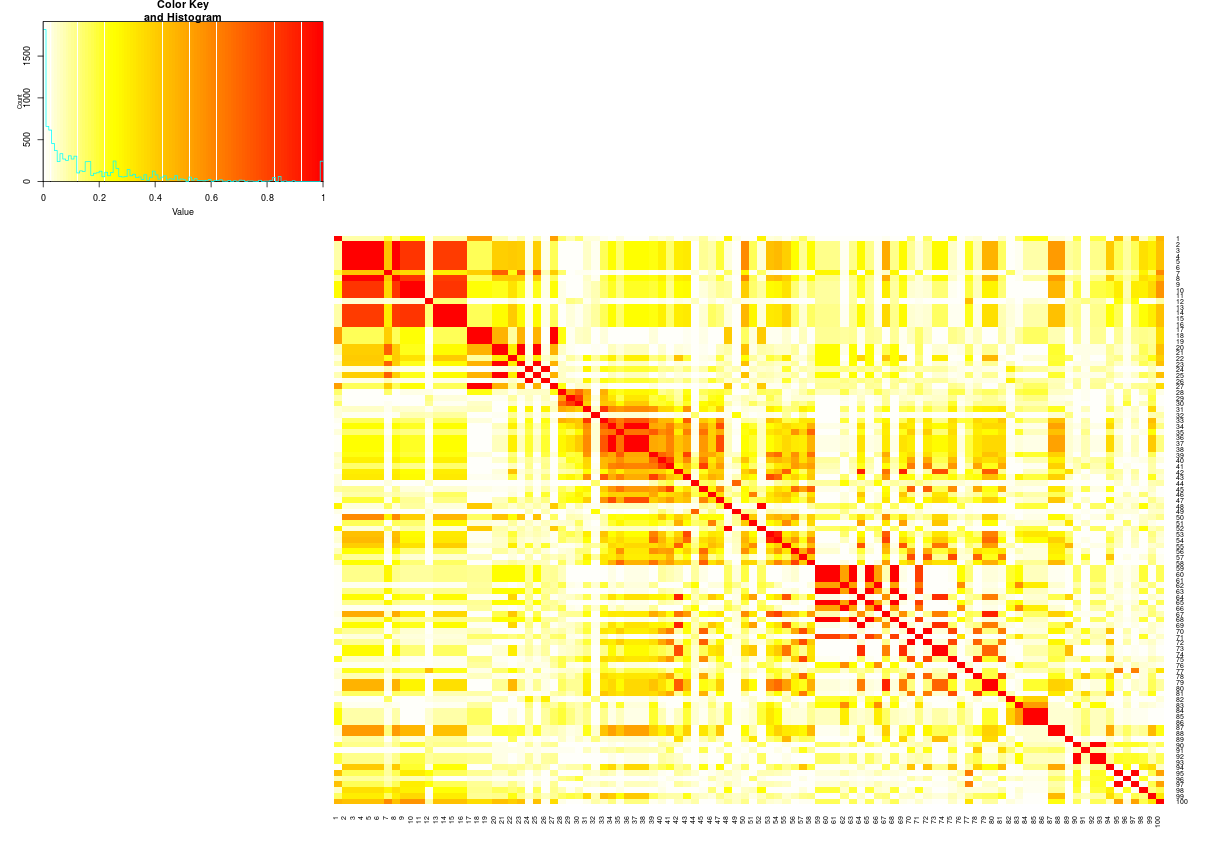## Introduction and examples for sim1000G

### Installing the package and dependencies (if installing from source)

Typically you can install sim1000G from the CRAN repository:

``````install.packages("sim1000G")
``````

The genetic map in use by the package is from the Hapmap 2010 release, lifted over to GrCH37 coordinates. It was downloaded from:

ftp://ftp.ncbi.nlm.nih.gov/hapmap/recombination/2011-01_phaseII_B37/

### Reading a VCF file and starting the simulation

Before starting the simulator, a VCF file of the region of interest is needed. The VCF file is used to provide the haplotypes that will be used for the simulator.

For this example we use an unfiltered region from 1000 genomes Phase III sequencing data VCF, chromosome 4, CEU samples.

We also need to initialize all the simulator internal structures with the command startSimulation.

The following parameters can be set:

• maxNumberOfVariants : total number of variants from the area that will be kept, generally should be < 1000
• min_maf : minimum allele frequency of the markers to keep. It should be >0.
• max_maf : maximum allele frequency of markers to keep
• maximumNumberOfIndividuals : how many simulated individuals to reserve space for
``````library(sim1000G)

examples_dir = system.file("examples", package = "sim1000G")
vcf_file = file.path(examples_dir, "region.vcf.gz")

vcf = readVCF( vcf_file , maxNumberOfVariants = 100 , min_maf = 0.02 , max_maf = NA )

startSimulation( vcf )
``````

## Generating unrelated individuals

Generation of new founder individuals is done using the function SIM\$addUnrelatedIndividual(). The function return the index of the individual generated.

After the individual is generated, its haplotypes are available at the arrays `SIM\$gt1[i,]` and `SIM\$gt2[i,]`.

An example with 30 individuals is below

``````# The following function will erase all the indivudals that have been simulated and start over.
# SIM\$reset()

id = c()
for(i in 1:30) id[i] = SIM\$addUnrelatedIndividual()

# Show haplotype 1  of first 5 individuals
#print(SIM\$gt1[1:5,1:6])

# Show haplotype 2
#print(SIM\$gt1[1:5,1:6])

genotypes = SIM\$gt1[1:20,] + SIM\$gt2[1:20,]

print(dim(genotypes))

str(genotypes)

library(gplots)

heatmap.2(cor(genotypes)^2, col=rev(heat.colors(100)) , trace="none",Rowv=F,Colv=F)
``````## Simulating genotypes within families

Simulationg of related individuals in a pedigrees requires modelling recombination events.

Below we show an example on how to simulate 100 families with 2 offspring each.

In addition we write the output to a PED/MAP file in plink format, for further analysis.

``````# Simulate one family with 2 offspring

fam = newFamilyWithOffspring("fam1",2)
print(fam)

# Simulate 100 families

SIM\$reset()

## For testing the IBD, we set the cM so that the regions spans to 4000cm
## Remove for normal use
SIM\$cm = seq( 0,4000, length = length(SIM\$cm) )

time10families = function() {

fam = lapply(1:10, function(x) newFamilyWithOffspring(x,2) )
fam = do.call(rbind, fam)
fam

}

fam <- time10families()

# Uncomment the following line to write a plink compatible ped/map file

# writePED(vcf, fam, "out")
``````

## Computing the IBD matrices

The simulator tracks the locations of all the ancestral alleles in 2 seperate arrays. These can be used to compute the IBD1,2 matrices, in arbitrary pedigrees.

Unfortunately, tracking the ancestral alleles makes the simulator a lot slower, so if we don't need this functionality, we can remove it later.

``````n = SIM\$individuals_generated

IBD1matrix =
sapply(1:n, function(y) {
z = sapply(1:n, function(x) computePairIBD12(x,y) )
names(z) = 1:n
z
})

IBD2matrix =
sapply(1:n, function(y) {
z = sapply(1:n, function(x) computePairIBD12(x,y) )
names(z) = 1:n
z
})
``````

### IBD1 matrix

``````colnames(IBD1matrix) = 1:nrow(IBD1matrix)
rownames(IBD1matrix) = 1:nrow(IBD1matrix)
colnames(IBD2matrix) = 1:nrow(IBD2matrix)
rownames(IBD2matrix) = 1:nrow(IBD2matrix)

knitr::kable(IBD1matrix[1:8,1:8] )
``````
1 2 3 4 5 6 7 8
0 0 1.00 1.00 0 0 0.0 0.0
0 0 1.00 1.00 0 0 0.0 0.0
1 1 0.00 0.47 0 0 0.0 0.0
1 1 0.47 0.00 0 0 0.0 0.0
0 0 0.00 0.00 0 0 1.0 1.0
0 0 0.00 0.00 0 0 1.0 1.0
0 0 0.00 0.00 1 1 0.0 0.6
0 0 0.00 0.00 1 1 0.6 0.0

### IBD2 matrix

``````colnames(IBD1matrix) = 1:nrow(IBD1matrix)
rownames(IBD1matrix) = 1:nrow(IBD1matrix)
colnames(IBD2matrix) = 1:nrow(IBD2matrix)
rownames(IBD2matrix) = 1:nrow(IBD2matrix)

knitr::kable(IBD2matrix[1:8,1:8] )
``````
1 2 3 4 5 6 7 8
1 0 0.00 0.00 0 0 0.00 0.00
0 1 0.00 0.00 0 0 0.00 0.00
0 0 1.00 0.31 0 0 0.00 0.00
0 0 0.31 1.00 0 0 0.00 0.00
0 0 0.00 0.00 1 0 0.00 0.00
0 0 0.00 0.00 0 1 0.00 0.00
0 0 0.00 0.00 0 0 1.00 0.16
0 0 0.00 0.00 0 0 0.16 1.00

## Simulating data in multi-generational pedigrees

The function to generate family data can be extended to simulate arbitraty pedigrees, it is shown below:

``````newFamilyWithOffspring = function(familyid, noffspring = 2) {

fam = data.frame(fid = familyid  ,
id = c(1:2) ,
father = c(0,0),
mother = c(0,0),
sex = c(1,2)
)

fam\$gtindex = c(j1,j2) # Holds the genotype position in the arrays SIM\$gt1 and SIM\$gt2

for(i in 1:noffspring) {
j3 = SIM\$mate(j1,j2)

newFamilyMember = c(familyid, i+10, 1,2, 1 , j3)
fam = rbind(fam, newFamilyMember)
}

return (fam)
}
``````

## Simulating a 3 generational pedigree and computing IBD1/2 matrices

In this example, we generate a pedigree with 6 individuals, across 3 generations. After that, we compute the IBD matrices of the family.

``````# Reset simulation
SIM\$reset()

# Set the region size in cM (0-4000cm, for testing the correctness of the function)
SIM\$cm = seq(0,4000,l=length(SIM\$cm))

C = SIM\$mate(A,B)
D = SIM\$mate(A,B)
E = SIM\$mate(G,C)

computePairIBD12(C,D)
computePairIBD12(E,A)

n = SIM\$individuals_generated

IBD1matrix =
sapply(1:n, function(y) {
z = sapply(1:n, function(x) computePairIBD12(x,y) )
names(z) = 1:n
z
})

IBD2matrix =
sapply(1:n, function(y) {
z = sapply(1:n, function(x) computePairIBD12(x,y) )
names(z) = 1:n
z
})

printMatrix(IBD1matrix)
``````
``````##    user  system elapsed
##   0.226   0.705   0.090
## IBD1 IBD2
## 0.56 0.19
## IBD1 IBD2
## 0.55 0.00
##          [   1]  [   2]  [   3]  [   4]  [   5]  [   6]
## [   1]    0.000   0.000   1.000   1.000   0.000   0.550
## [   2]    0.000   0.000   1.000   1.000   0.000   0.450
## [   3]    1.000   1.000   0.000   0.560   0.000   1.000
## [   4]    1.000   1.000   0.560   0.000   0.000   0.460
## [   5]    0.000   0.000   0.000   0.000   0.000   1.000
## [   6]    0.550   0.450   1.000   0.460   1.000   0.000
``````